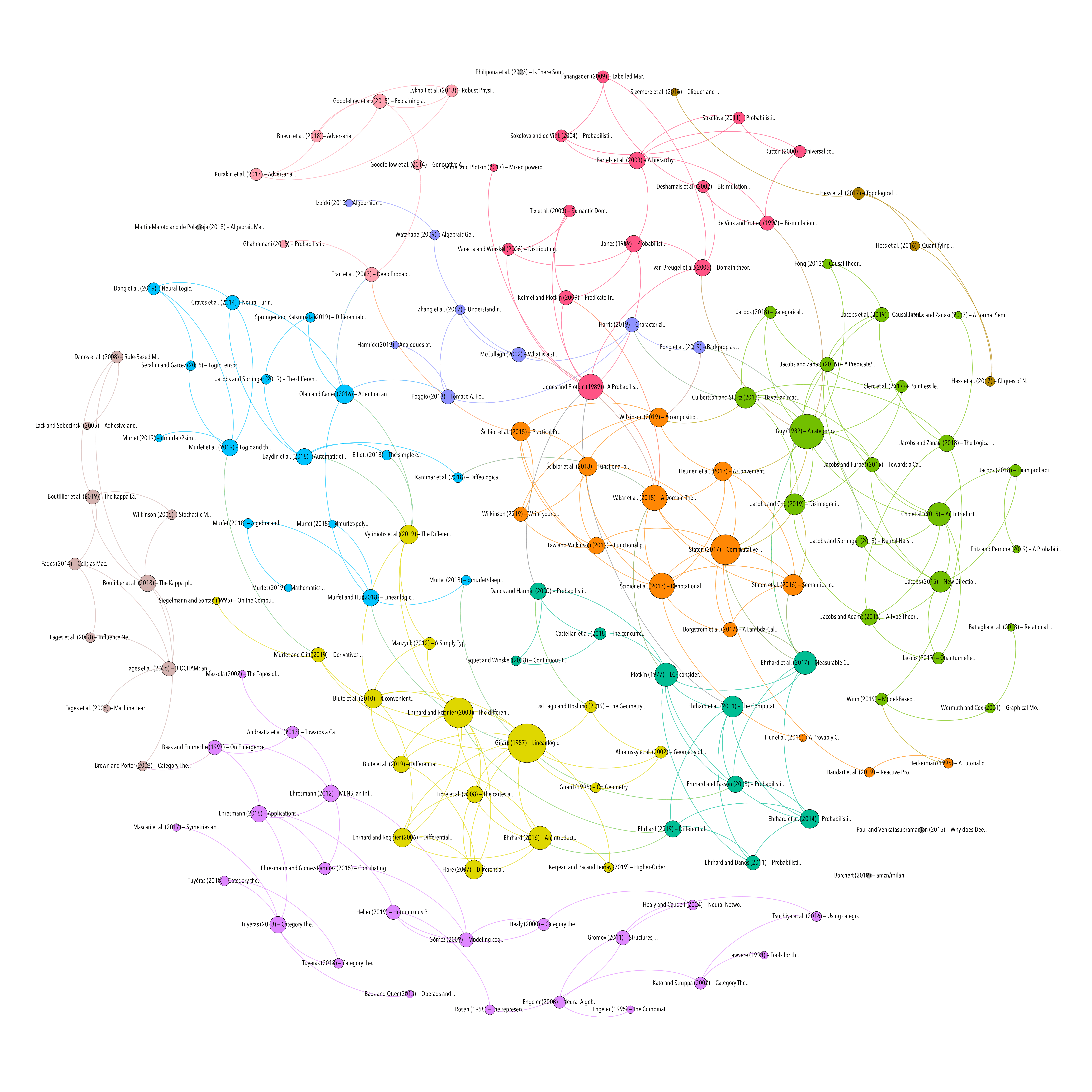CATEGORICAL LOGIC

Geometry of Interaction and Linear Combinatory Algebras

Resource type
Authors/contributors
Title
Geometry of Interaction and Linear Combinatory Algebras
Abstract
We present an axiomatic framework for Girard's Geometry of Interaction based on the notion of linear combinatory algebra. We give a general construction on traced monoidal categories, with certain additional structure, that is sufficient to capture the exponentials of Linear Logic, which produces such algebras (and hence also ordinary combinatory algebras). We illustrate the construction on six standard examples, representing both the ‘particle-style’ as well as the ‘wave-style’ Geometry of Interaction.
Publication
Mathematical. Structures in Comp. Sci.
Volume
12
Issue
5
Pages
625–665
Date
October 2002
DOI
10/fcsmhm
ISSN
0960-1295
Accessed
2019-11-28T15:33:04Z
Library Catalog
ACM Digital Library
Extra
ZSCC: 0000162
Citation
Abramsky, S., Haghverdi, E., & Scott, P. (2002). Geometry of Interaction and Linear Combinatory Algebras. Mathematical. Structures in Comp. Sci., 12(5), 625–665. https://doi.org/10/fcsmhm
CATEGORICAL LOGIC
Topic
Processing time: 0.02 secondsGraph of references (from Zotero to Gephi via Zotnet with this script)